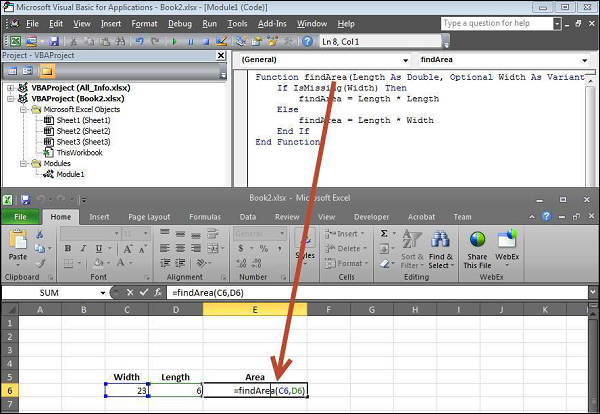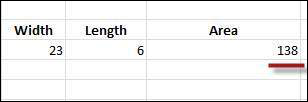# VBA - User Defined Functions

A function is a group of reusable code which can be called anywhere in your program. This eliminates the need of writing the same code over and over again. This enables the programmers to divide a big program into a number of small and manageable functions.

Apart from inbuilt functions, VBA allows to write user-defined functions as well. In this chapter, you will learn how to write your own functions in VBA.

## Function Definition

A VBA function can have an optional return statement. This is required if you want to return a value from a function.

For example, you can pass two numbers in a function and then you can expect from the function to return their multiplication in your calling program.

Note − A function can return multiple values separated by a comma as an array assigned to the function name itself.

Before we use a function, we need to define that particular function. The most common way to define a function in VBA is by using the Function keyword, followed by a unique function name and it may or may not carry a list of parameters and a statement with End Function keyword, which indicates the end of the function. Following is the basic syntax.

### Syntax

```Function Functionname(parameter-list)
statement 1
statement 2
statement 3
.......
statement n
End Function
```

### Example

Add the following function which returns the area. Note that a value/values can be returned with the function name itself.

```Function findArea(Length As Double, Optional Width As Variant)
If IsMissing(Width) Then
findArea = Length * Length
Else
findArea = Length * Width
End If
End Function
```

## Calling a Function

To invoke a function, call the function using the function name as shown in the following screenshot.The output of the area as shown below will be displayed to the user.